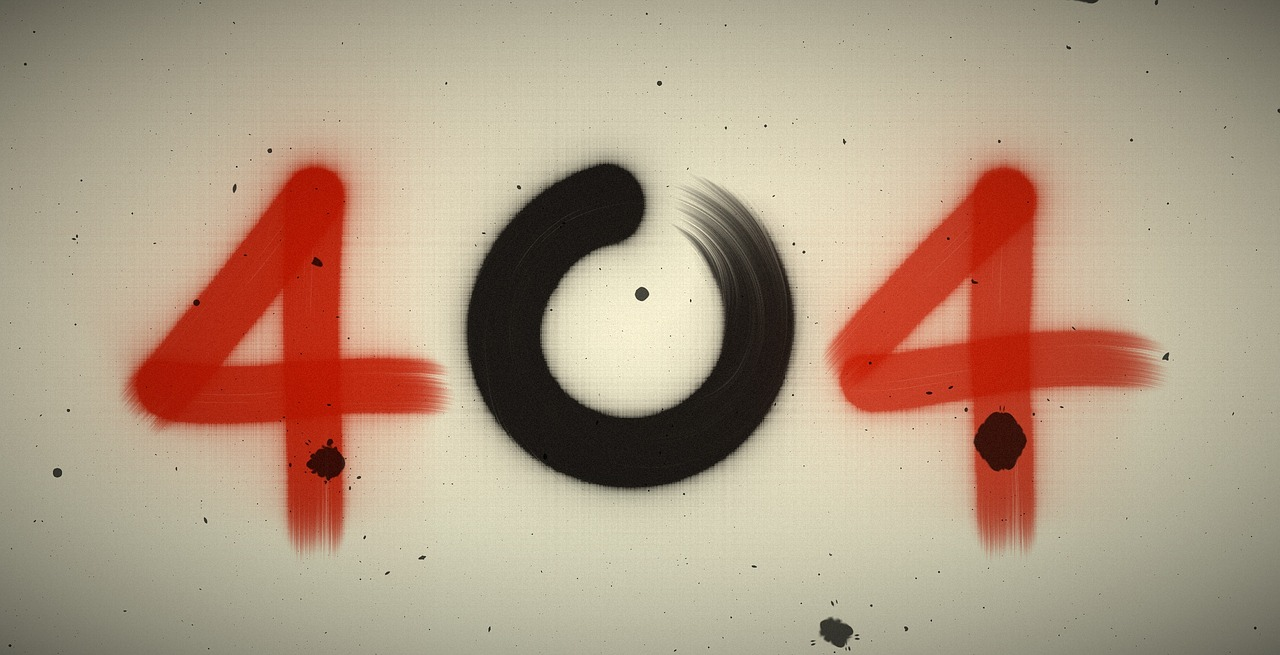## Post per Page

A mathematician has solved a 30-year-old problem at the boundary between mathematics and computer science. He used an innovative, elegant proof that has his colleagues marveling at its simplicity.

Hao Huang, an assistant professor of mathematics at Emory University in Atlanta, proved a mathematical idea called the sensitivity conjecture, which, in incredibly rough terms, makes a claim about how much you can change the input to a function without changing the output (this is its sensitivity).

In the decades since mathematicians first proposed the sensitivity conjecture (without proving it), theoretical computer scientists realized that it has huge implications for determining the most efficient ways to process information .

What did Huang actually prove?

For simplicity's sake, imagine a 3D cube with sides that are each 1 unit long. If you put this cube into a 3D coordinate system (meaning it has measurements in three directions), one corner would have the coordinates (0,0,0), the one next to it might be (1,0,0), the one above it might be (0,1,0) and so on. You can take half the corners (four corners) without having any pair of neighbors: (0,0,0) , (1,1,0), (1,0,1) and (0,1,1) aren't neighbors. You can show this by looking at the cube, but we also know it because all of them are different by more than one coordinate.

The sensitivity conjecture is about finding how many neighbors you have when you take more than half the corners of a higher dimensional cube, or a hypercube, said Hebrew University mathematician Gil Kalai. You can write the coordinates of the hypercube as strings of 1s and 0s, where the number of dimensions is the length of the string, Kalai told Live Science. For a 4D hypercube, for instance, there are 16 different points, which means 16 different strings of 1s and 0s that are four digits long.

Now pick half plus 1 individual points on the hypercube (for a 4D hypercube, that means pick nine — or 8+1 — different points out of a total of 16). [Mathematicians Edge Closer to Solving a 'Million Dollar' Math Problem]

From this smaller set, find the point with the most neighbors — what's the minimum number of neighbors it can have? (Neighbors differ by just one number. For example, 1111 and 1110 are neighbors, because you only have to swap one digit to turn the first into the second.)

Huang proved that this corner must have at least as many neighbors as the square root of the number of digits — in this case, the square root of 4 — which is 2.

For low dimensions, you can tell this is true just by checking. It's not that hard to check 16 coordinates on the cube (or "strings") for neighbors, for example. But every time you add a dimension to the cube, the number of strings doubles. So the problem gets harder to check very quickly. [A Mathematician Just Solved a Deceptively Simple Puzzle That Has Boggled Minds for 64 Years]

The set of strings that's 30 digits long — the coordinates to the corners of a 30-dimensional cube — has more than 1 billion different strings in it, meaning the cube has more than 1 billion corners. With strings that are 200 digits long, there are more than a novemdecillion. That's a million billion billion billion billion billion billion, or 1 followed by 60 zeroes.

This is why mathematicians like proofs: They show that something is true in every case, not just the easy ones.

"If n is equal to a million — this means we have strings of length 1 million — then the conjecture is that if you take 2^1,000,000-1 and add 1, then there is a string that has 1,000 neighbors — the square root of a million," Kalai said.

The last major advance in the sensitivity conjecture came in 1988, Kalai said, when researchers proved that one string has to have at least the logarithm of n neighbors. That's a much lower number; the logarithm of 1,000,000 is just 6. So Huang's proof just discovered that at least 994 other neighbors are out there.

An elegant and "mysterious" proof

"It is very mysterious," Kalai said of Huang's proof. "It uses 'spectral methods,' which are very important methods in many areas of mathematics. But it uses spectral methods in a novel way. It's still mysterious, but I think we can expect that this novel way to use spectral methods will gradually have more applications."

In essence, Huang conceptualized the hypercube using arrays of numbers in rows and columns (called matrices). Huang figured out a completely unexpected way to manipulate a matrix with an unusual arrangement of -1s and 1s that "magically makes it all work," Aaronson wrote on his blog. [10 Surprising Facts About Pi]

Huang "took this matrix, and he modified it in a very ingenious and mysterious way," Kalai said. "It's like you have an orchestra and they play some music, and then you let some of the players, I don't know, stand on their head, and the music becomes completely different — something like that."

That different music turned out to be the key to proving the conjecture, Kalai said. It's mysterious, he said, because even though mathematicians understand why the method worked in this case, they don't fully understand this new "music" or in what other cases it might be useful or interesting.

"For 30 years, there was no progress, and then Hao Huang settled this problem, and he found a very simple proof that the answer is the square root of n," Kalai said. "But during these 30 years … people realized that this question is very important in the theory of computing."

Huang's proof is exciting because it advances the field of computer science, Kalai said. But it's also noteworthy because it introduced a novel method, and mathematicians still aren't sure what else Huang's new method might allow them to accomplish.